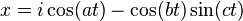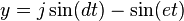Actions

# Plotting parametric equations¶

Let's plot some parametric equations, with a small twist. This is basically the
core of ParametricFun, and it was to that end that this class was created. For
this example we'll use some easier equation.

In order to obtain some parametric equations, let's checkout Wikipedia.

For this example we'll be plotting:## Import the classes¶

``````import com.dkt.graphics.extras.formula.ParametricCalculable;
import com.dkt.graphics.extras.formula.ParametricCalculableTimer;
``````

## Declare variables¶

Since this equations have a lot of parameters, we'll declare some auxiliary variables to clarify the code a bit.

``````// This values will change the output as explained
// in the Wikipedia article, so change them at will
final double a = 1;
final double b = 60;
final double c = 1;
final double d = 1;
final double e = 60;
final double i = 1;
final double j = 2;
``````

## Create a new `ParametricCalculable`¶

We will create a new `ParametricCalculable` object, and implement `x(t)` and `y(t)` according to the specified equation, in this case:

``````ParametricCalculable eq = new ParametricCalculable() {
@Override
public double x(double t) {
return i * Math.cos(a*t) - Math.cos(b*t) * Math.sin(c*t);
}

@Override
public double y(double t) {
return j * Math.sin(d*t) - Math.sin(e*t);
}
};
``````

## Edit the drawing and calculation parameters¶

As before we need to scale the equation, and set the interval and step:

``````eq.setScaleX(80);
eq.setScaleY(80);
eq.startPoint(0);
eq.endPoint(2 * Math.PI);
eq.step(0.000001);
``````

## Create a `ParametricCalculableTimer`¶

Since this is a timer, we'll use the auto repaint feature of the canvas.

``````canvas.setAutoRepaint(true);
canvas.setRepaintDelay(25);
``````

And finally create a new timer and run it!

``````ParametricCalculableTimer timer = new ParametricCalculableTimer(eq);
timer.start();
``````

## Misc¶

This class has some interesting features that I invite you to play with:

• `ParametricCalculableTimer#setNumberOfThreads(...)`
• `ParametricCalculableTimer#setDrawPen(...)`
• `ParametricCalculableTimer#setDrawAsPath(...)`
• `ParametricCalculableTimer#pause()`
• `ParametricCalculableTimer#resume()`

There are some situations where you'll need to take some action when the timer starts, pauses, resumes or ends; to that end there's an interface that you can use `AbstractTimer.Action` and then register it in your timer with something like:

``````ParametricCalculableTimer#setActions(new AbstractTimer.Action() {
@Override
public void start() {
}
@Override
public void pause() {
}
@Override
public void resume() {
}
@Override
public void stop() {
}
});
``````

This comes in handy when dealing with the auto repaint feature, since we can use it to show an "animation" of the equation being calculated, but we need to cancel it once the calculation has finished. On the other hand, if we only care about the end result, it's much faster to calculate without drawing, and draw all together once all the threads are done.

The end result should look something like this:The final code can be downloaded from here `->` Parametric.java

Updated by Federico Vera almost 3 years ago · 3 revisions

Go to top